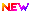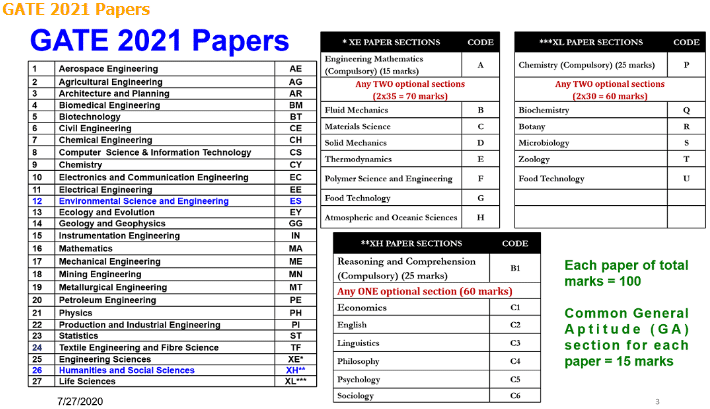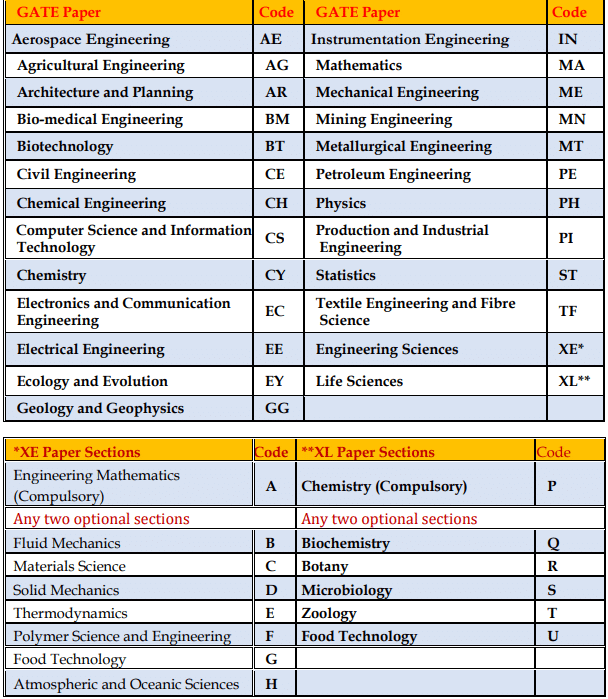GATE Exam Pattern 2022, Paper Pattern, Marking Scheme – Check Here

Latest Applications Open 2022:GATE 2022 Examination Schedule Details will be released in March 2021. GATE stands for (Graduate Aptitude Test Engineering) is a national-level engineering entrance examination. GATE is conducted jointly by the Indian instituted of Science and seven Indian Institutes of Technology (Bombay, Delhi, Guwahati, Kanpur, Kharagpur, Madras, and Roorkee) on behalf of the National Coordination Board. From this year, the GATE 2022 Examination will be conducted by IIT Bombay.

GATE 2022 Exam will be conducted in February 2022. All 27 Papers will be conducted through Computer Based Test. From the Year 2022, 2 more Subjects have been added a total of 27. GATE 2022 Exam pattern has been announced by the IIT Bombay. GATE 2022 exam pattern article consists of complete information about the GATE 2022 exam pattern.

GATE Exam is conducted as an entrance exam for programming in engineering at the national level. Study materials for GATE are available online for all branches. For the GATE 2022 Examination, a candidate can apply for only one of the 27 Papers.

GATE 2022 Examination – Schedule Details ReleasedGATE Previous Year Examination Schedule Details has been Released now. Click Here to View Examination Schedule.Since the Year 2021, The New Subjects is Added with two new subjects — Environmental Science and Engineering, or ES, and Humanities and Social Science, or XS. Now total Subjects will 27th. Click Here to View Notice.

There are three sections in the GATE paper for AE, AG, AR, BT, BM, CE, CH, CS, CY, EC, EE, ES, IN, ME, MN, MT, PI, TF, XE, AR, CY GG, MA, PH, XH, and XL.

• Subject question
• Engineering Mathematics / Chemistry
• General AptitudeGATE Exam Pattern 2022

Latest Applications For Various UG & PG Courses Open 2021:

1. UPES, Dehradun | Admissions Open for All Courses 2022. Apply Now
 Feature Description Exam Duration 3 Hours (180 minutes) Number of Questions 65 Total Marks 100 Question Types Two types of questions asked: Multiple Choice Questions (MCQ) Numerical Answer Type (NAT) Questions Marking Scheme For 1-mark MCQs, 1/3 mark will be deducted for every incorrect attempt. In the case of 2-mark MCQs, the candidate will be penalized 2/3 mark for the wrong attempt For questions that aren’t attempted, zero marks will be awarded. There is no negative marking for numerical answer type (NAT) questions. Sections The question paper of GATE 2022 will consist of three sections: General Aptitude Engineering Mathematics (for XL papers it is Chemistry) Subject-specific section Mode Online Sectional Time-Limit No
Candidates will be permitted to occupy their allotted seats 40 minutes before the scheduled start of the examination.
Candidates can log in and start reading the instructions 20 minutes before the start of the examination.
Candidates will NOT be permitted to enter the examination hall after 10:00
hours in the forenoon session and after 15:00 hours in the afternoon session. Candidates will NOT be permitted to leave the examination hall before the end of the examination.

*General Aptitude & Engineering Mathematics will be mandatory.

General Aptitude:

Each paper will include a mandatory General Aptitude (GA) section comprising 10 questions. 5 of these questions will carry 1-mark each, while the remaining 5 will each carry 2 marks, for a total of 15 marks.

In all the papers, General Aptitude (GA) there will be a total of 65 questions carrying 100 marks, out of which ten questions are giving a total of 15 marks.

In the papers bearing the codes AE, AG, BM, BT, CE, CH, CS, EC, EE, IN, ME, MN, MT, PE, PI, TF and XE, the Engineering Mathematics will carry around 15% of the total marks, the General Aptitude section will carry 15% of the total marks and the remaining 70% of the total marks is devoted to the subject of the paper.In the papers bearing the codes AR, CY, EY, GG, MA, PH, and XL, the General Aptitude section will carry 15% of the total marks, and the remaining 85% of the total marks is devoted to the subject of the paper.

*XE (Engineering Sciences) and **XL (Life Sciences) papers are general and will be comprised of Sections listed in the above table.

Note: CE and ME papers: Examination may be conducted in multiple sessions. However, the candidate is allowed to appear in only ONE specified session.

Questions GATE 2022

Multiple Choice Questions (MCQ) carrying 1 or 2 marks each in all articles and sections. These issues are objective, and each will have a choice of four answers, out of which the candidate has to mark the correct answer(s).

(NAQ) Numerical Answer Questions of 1 or 2 marks each in all papers and sections. For these questions the answer is a real number, to be entered by the candidate using the virtual keypad. No choices will be shown for this type of question.

The answer can be a number such as 10 (an integer only). The answer may be in decimals as well, for example, 10.1 (one decimal) or 10.01 (two decimals), or 10.001 (three decimals). Wherever required and possible, it is better to give NAT answers up to a maximum of three decimal places.

Negative Marketing

• For the multiple-choice questions (MCQs) there would be negative marking for the wrong answer.
• For one mark multiple-choice questions, 1/3 mark will be deducted for a wrong answer.
• For two mark multiple-choice questions, a 2/3 mark will be deducted for a wrong answer.
• There is NO negative marking for numerical answer-type questions.

General Aptitude (GA) Questions

In all papers, GA questions carry a total of 15 marks. The GA section includes five questions carrying 1 – mark each (sub-total five marks) and five questions carrying 2 – marks each (sub-total 10marks).

XE Paper

 Section Type of Question Number of Questions Sub-total (marks) Section A (Compulsory) 1-mark questions 7 7 2-mark questions 4 8 Sections B to H 1-mark questions 9 9 2-mark questions 13 26

These documents would contain 25 questions carrying 1 – mark each (sub-total 25 marks) and 30 questions carrying 2 – marks each (sub-total 60 marks). The question paper will consist of questions of multiple choice and numerical answer type. For mathematical respond to questions, choices will not be given.

Candidates have to enter the answer (which will be a real number, signed or unsigned, e.g., 25.06, – 25.06, 25, – 25, etc.) using a virtual keypad.

The questions shall be mentioned with up to which decimal places, the candidates need to make an answer. Also, an appropriate range will be considered while evaluating the numerical answer type questions so that the candidate is not penalized due to the usual round–off errors.

GG (Geology & Geophysics) Paper

Apart from the General Aptitude (GA) section, the GG question paper consists of two parts: Part A and Part B. Part A are common for all candidates.

Part B contains two sections: Section 1 (Geology) and Section 2 (Geophysics). Candidates will have to attempt questions in Part A and either Section 1 or Section 2 in Part B.

Part A consists of 25 multiple–choice questions carrying 1 – mark each (sub-total 25 marks and some of these may be numerical answer type questions). Each section in Part B (Section 1 and Section 2) consists of 30 multiple choice questions carrying 2 – marks each (sub-total 60 marks and some of these may be numerical answer type questions).

XE Paper (Engineering Sciences)

In the XE paper, the Engineering Mathematics section (Section A) is compulsory. This section contains 11 questions carrying a total of 15 marks: 7 questions carrying 1 – mark each (sub-total seven marks), and four issues carrying 2 – marks each (sub-total eight marks). Some questions may be of numerical answer type questions.

Each of the other sections of the XE paper (Sections B through H) contains 22 questions carrying a total of 35 marks: 9 questions carrying 1 – mark each (sub-total nine marks) and 13 questions carrying 2 – marks each (sub-total 26 marks). Some questions may be of numerical answer type.

GATE Exam Pattern – XL Paper

 Section Type of Question Number of Questions Sub-total (marks) Chemistry Section or Section P (Compulsory) 1-mark questions 5 5 2-mark questions 10 20 Sections Q to U 1-mark questions 10 10 2-mark questions 10

In XL paper, the Chemistry section (Section P) is compulsory. This section contains 15 questions carrying a total of 25 marks: 5 questions carrying 1 – mark each (sub-total five marks)and ten questions carrying 2 – marks each (sub-total 20 marks). Some questions may be of numerical answer type

Each of the other sections of the XL paper (Sections Q through U) contains 20 questions carrying a total of 30 marks: 10 questions carrying 1 – mark each (sub-total ten marks) and ten questions carrying 2 – marks each (sub-total 20 marks). Some questions may be of numerical answer type.

GATE 2022 Exam Paper Codes for XE and XL papers

 XE Paper Sections Code XL Paper Sections Code Engineering Mathematics (Compulsory) A Chemistry (Compulsory) P Fluid Mechanics B Biochemistry Q Materials Science C Botany R Solid Mechanics D Microbiology S Thermodynamics E Zoology T Polymer Science and Engineering F Food Technology U Food Technology G Atmospheric and Oceanic Sciences H

GATE 2022 Paper Analysis

Each paper will also include General Aptitude questions attempted. The subject-specific questions are assigned according to the preference of the contenders. The total marks for the examination will be 100. GATE 2022 Exam pattern permits the applicants to be acquainted with the study to be organized so that they prepare appropriately.

Gate 2022 Paper Analysis for In by The Gate Academy

Latest Applications For Various UG & PG Courses Open 2021:

1. UPES, Dehradun | Admissions Open for All Courses 2022. Apply Now

Applicants can check the GATE paper analysis 2022 representing IN by The GATE Academy at this juncture. GATE 2022 paper analysis for IN by The GATE Academy assists contenders to get an inkling about the exam difficulty level, type of questions asked, weightage given to each theme, trends, and evaluations with previous year papers. This year GATE is held at 199 cities throughout India and 6 cities abroad.

If candidates have any queries regarding GATE 2022 Exam Pattern, they can leave their comments below.

1.Madhusmita patra says:

Good